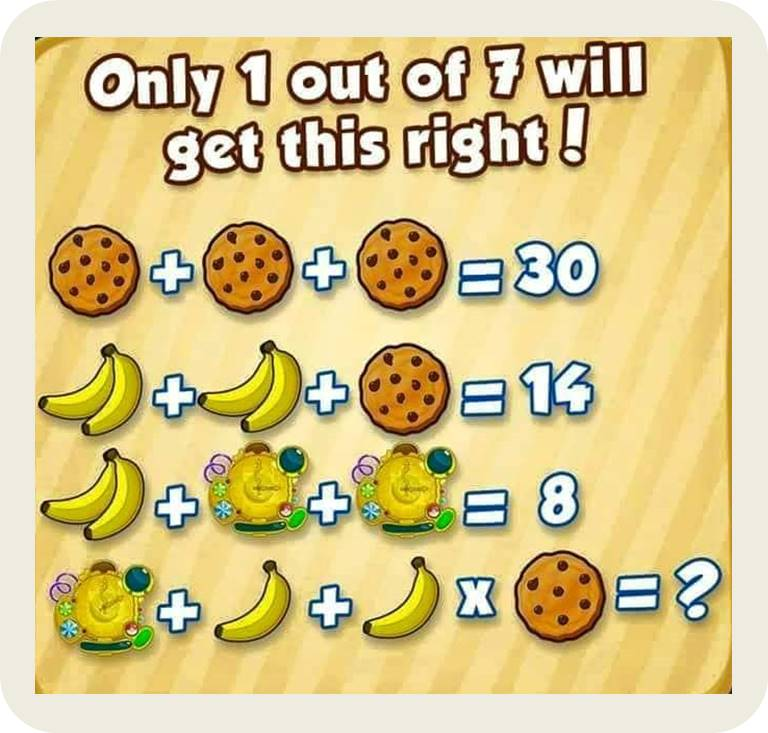# Datarmatics Researchers Decimate Biscuit-and-Banana PuzzleDr. Wonu Nduka
Mathematically!😁

Let the biscuit be “x”
let banana be “y” and
Let lollipop be “z”

Such that the pictorial equations can be transformed into algebraic equations,  thus:

x+x+x=30…..(1)
y+y+x=14…..(2)
y+z+z=8…….(3)
‘z’+0.5y+0.5y’x’=?…..(4)

Equations 1, 2 &3 could further be reduced to:

3x=30……..(5)
x+2y=14….(6)
y+2z=8……(7)

From equation (5) we have:
3x=30
x=10
substitute x=10 in equation (6)
2y+10=14
2y+10-10=14-10
2y=4
y=2

Now, substitute y=2 in equation (7)
y+2z=8
2-2+2z=8-2
2z=6
z=3

To evaluate the expression (4), where x=10, y=2 and z=3.
This is the overarching part of the problem-solving episode.

Considering the time, the fingers of the banana and the number of dots on the biscuits.

If x=10 when dots were 10, then x=7, when dots became 7.

If y=2 when we had two fingers of banana, then y=1 when the fingers of banana reduced to 1.

If z=3, when the time was 3 o’clock, then z=2, when the time became 2 o’clock.

When the logic above is being applied to the expression (4) we have:
👇
y+yx+z
1+1*7+2
1+7+2=10

EVALUATION
Check using the previous equations, with x=10, y=2 and z=3

x+x+x=30…..(1)
y+y+x=14…..(2)
y+z+z=8…….(3)

From equation (1)
10+10+10=30✅

From equation (2)
2+2+10=14✅

From equation (3)
2+3+3=8✅

Prof. Ebi Efebo
We need to think outside the box
Each of the biscuits have 10 dots
3×10=30
Each dot represents 1
2nd equation
Each finger of banana is  1
2+ 2+10=14
Equation 3
2+3+3=8
2 fingers of banana and the 2 clocks at 3 o’clock  each
Equation 4
2 o’clock+ 1 finger of banana + another finger of banana × 7 dots on the biscuits
2+1+1×7
Applying BODMAS
7×1+1+2
7+1+2=10
That is the answer